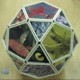# Period Panes

In The Penguin Dictionary of Curious and Interesting Numbers (1987), David Wells remarks that 142857 is “a number beloved of all recreational mathematicians”. He then explains that it’s “the decimal period of 1/7: 1/7 = 0·142857142857142…” and “the first decimal reciprocal to have maximum period, that is, the length of its period is only one less than the number itself.”

Why does this happen? Because when you’re calculating 1/n, the remainders can only be less than n. In the case of 1/7, you get remainders for all integers less than 7, i.e. there are 6 distinct remainders and 6 = 7-1:

(1*10) / 7 = 1 remainder 3, therefore 1/7 = 0·1...
(3*10) / 7 = 4 remainder 2, therefore 1/7 = 0·14...
(2*10) / 7 = 2 remainder 6, therefore 1/7 = 0·142...
(6*10) / 7 = 8 remainder 4, therefore 1/7 = 0·1428...
(4*10) / 7 = 5 remainder 5, therefore 1/7 = 0·14285...
(5*10) / 7 = 7 remainder 1, therefore 1/7 = 0·142857...
(1*10) / 7 = 1 remainder 3, therefore 1/7 = 0·1428571...
(3*10) / 7 = 4 remainder 2, therefore 1/7 = 0·14285714...
(2*10) / 7 = 2 remainder 6, therefore 1/7 = 0·142857142...

Mathematicians know that reciprocals with maximum period can only be prime reciprocals and with a little effort you can work out whether a prime will yield a maximum period in a particular base. For example, 1/7 has maximum period in bases 3, 5, 10, 12 and 17:

1/21 = 0·010212010212010212... in base 3
1/12 = 0·032412032412032412... in base 5
1/7 =  0·142857142857142857... in base 10
1/7 =  0·186A35186A35186A35... in base 12
1/7 =  0·274E9C274E9C274E9C... in base 17

To see where else 1/7 has maximum period, have a look at this graph:Period pane for primes 3..251 and bases 2..39

I call it a “period pane”, because it’s a kind of window into the behavior of prime reciprocals. But what is it, exactly? It’s a graph where the x-axis represents primes from 3 upward and the y-axis represents bases from 2 upward. The red squares along the bottom aren’t part of the graph proper, but indicate primes that first occur after a power of two: 5 after 4=2^2; 11 after 8=2^3; 17 after 16=2^4; 37 after 32=2^5; 67 after 64=2^6; and so on.

If a prime reciprocal has maximum period in a particular base, the graph has a solid colored square. Accordingly, the purple square at the bottom left represents 1/7 in base 10. And as though to signal the approval of the goddess of mathematics, the graph contains a lower-case b-for-base, which I’ve marked in green. Here are more period panes in higher resolution (open the images in a new window to see them more clearly):Period pane for primes 3..587 and bases 2..77Period pane for primes 3..1303 and bases 2..152

An interesting pattern has begun to appear: note the empty lanes, free of reciprocals with maximum period, that stretch horizontally across the period panes. These lanes are empty because there are no prime reciprocals with maximum period in square bases, that is, bases like 4, 9, 25 and 36, where 4 = 2*2, 9 = 3*3, 25 = 5*5 and 36 = 6*6. I don’t know why square bases don’t have max-period prime reciprocals, but it’s probably obvious to anyone with more mathematical nous than me.Period pane for primes 3..2939 and bases 2..302Period pane for primes 3..6553 and bases 2..602

Like the Ulam spiral, other and more mysterious patterns appear in the period panes, hinting at the hidden regularities in the primes.

## 2 thoughts on “Period Panes”

1.Coagulopath says:

The “spoke” patterns radiating out from the bottom left look cool.

I wonder if it’s possible to extend the picture downward as well as upward. Negative primes aren’t a thing but negative bases apparently exist – would they show a similar pattern (if any?). I’m not sure enough of what I’m doing to try it.

•Krilling for Company says:

Thanks — I hadn’t come across negative bases before, so I’ll have to look into it.

I’m not sure enough of what I’m doing to try it.

The math and programming are much easier than learning music.

This site uses Akismet to reduce spam. Learn how your comment data is processed.